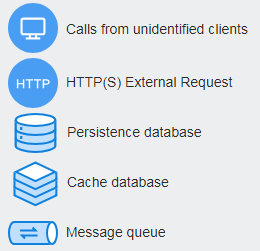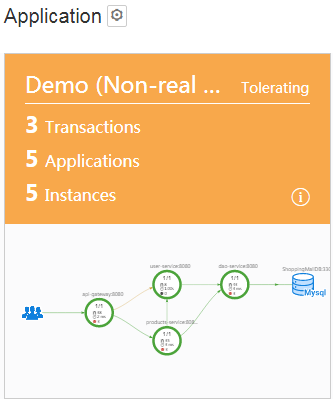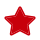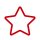Help Center> Application Performance Management> FAQs> Usage FAQs> How Do I Calculate the Number of Used Instances?
Updated on 2022-06-09 GMT+08:00

# How Do I Calculate the Number of Used Instances?

In Application Performance Management (APM), the number of used instances is calculated based on the number of probes. One probe corresponds to one service instance. You can obtain the number of used instances by calculating the number of probes. Note that the instances listed in Figure 1 do not use probes to report data and need to be excluded. The formula is as follows: Number of used instances (equals to that of probes) = Total number of instances on the application topology page – Number of instances that do not use probes

Figure 1 Instances that do not use probesExample of calculating the number of used instancesIn the preceding figure, there are five instances, one of which is the MySQL database.

Number of used instances (equals to that of probes) = 5 – 1 = 4

# Usage FAQs FAQs

more